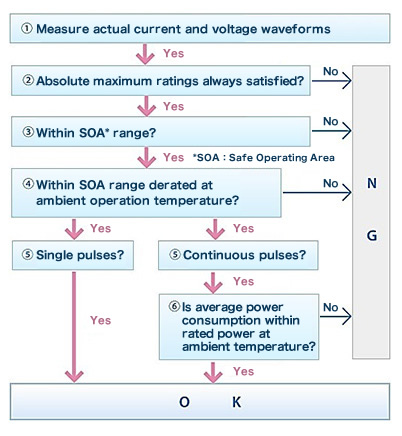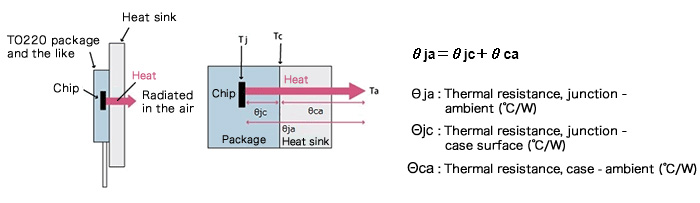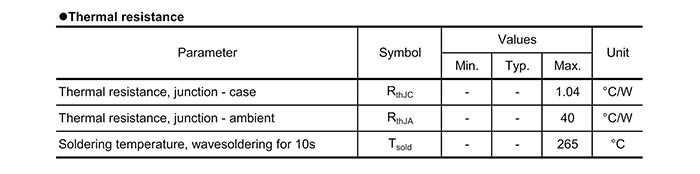# Confirmation of the Chip Temperature

2018.04.05

・It should be confirmed that Tj ultimately does not exceed the absolute maximum rating.

・Tj is the sum of Ta or Tc and the heat generation (the product of the thermal resistance and the power consumption).

In this chapter, we explain methods and procedures for determining whether or not a selected transistor is appropriate in actual operation.

Although not present in the flowchart on the right, in this section we discuss ⑦ in the list below, “Confirmation of the chip temperature”. In essence, once ⑥, “Confirmation that average power consumption is within the rated power” is completed, there are generally no problems, but safety can be further ensured with respect to the chip temperature.## ⑦Confirmation of the Chip Temperature

At the end of the previous section, “Confirmation that Average Power Consumption is within the Rated Power”, it was stated that “There are a number of methods for confirming that the power consumption is within the rated value, but they are in the end equivalent to determining whether Tj is within the absolute maximum rated value”. By way of follow-up, this time we discuss methods for determining the chip temperature, that is, Tj.

In actuality, if the power consumption is within the given rated value, then the chip temperature is below the absolute maximum rated value. This is because, as explained the last time, the power rating is calculated on the basis of the chip temperature. Hence whether usage conditions are appropriate or not can be judged through the confirmation steps described up till now. However, we have added the further step of confirming the chip temperature because, in addition to further heightening certainty, it serves as a firmer foundation when considering cases in which the given rated values are unfortunately exceeded.

One factor determining the chip temperature is the thermal resistance. Although this is explained below in terms of an equation, the relation is simple: when the thermal resistance is lowered, the chip temperature falls. In general, heat dissipation design is executed such that, within the constraints of the conditions of use, the required power is obtained while keeping the chip temperature Tj within the maximum rated value. The power that can be handled given the thermal resistance of a lone transistor is far lower than the performance of which the transistor is capable. In particular, a power transistor is generally used together with a heat sink. In the heat dissipation design for such a transistor, the chip temperature Tj is the most reliable parameter.

## What is the Chip Temperature?

To state the obvious, the chip temperature is the temperature of the transistor chip itself, represented by Tj (the junction temperature). The chip temperature Tj is the temperature resulting from addition of the ambient (surrounding environment) temperature Ta and the heat generated by the chip, and is one of the most important factors to consider when discussing chip ratings and lifetime.

## Calculation of the Chip Temperature

In recent years many transistors, such as the R6020ENZ used in examples, are resin-sealed.

Consequently, it is of course not possible to measure the chip temperature directly. Hence Tj is essentially determined through calculations. The equation used is as follows.

Tj＝Ta＋θja×P

※ Tj：Chip (junction) temperature, Ta：Ambient temperature（℃）, θja：Thermal resistance, junction to ambient（℃/W）, P：Power consumption（W）

As mentioned briefly above, and as the equation indicates, the chip temperature Tj is obtained by adding the ambient temperature Ta to the product of the thermal resistance and the power, that is, the chip heat generation. This is the most basic equation for Tj.

The diagram indicates the relationship between temperature and thermal resistance for different parts. The diagram includes a heat-dissipating plate (heat sink), but the case (package) temperature Tc is the temperature of the transistor package surface. The thermal resistances are related as follows.The thermal resistance θca when there is no heat sink is simply the result of subtracting θjc from the given value of θja. When a heat sink is present, the thermal resistance of the heat sink corresponds to θca, and conversely, θja is not simply the value given, but is the result of adding θjc and θca. The following is an excerpt from a data sheet. Nearly all power transistors use heat sinks, and so in general θja and θjc are given.In general, θjc is quite lower compared with θja, and so θja can be reduced considerably depending on the thermal resistance of the heat sink. θja itself (indicated by RthJA in the table) is 40°C/W, but if for example a heat sink with a thermal resistance of 10°C/W is used, then θja can be reduced to 11.04°C/W*. Moreover, when the heat sink is assumed to be infinite in expanse, it can be assumed that θja= θjc. (*The result of a simple calculation for purposes of illustration. An actual θca must be determined considering the thermal resistance at the junction between the package surface and the heat sink. Moreover, the thermal resistance of a heat sink is selected by calculating the value necessary for Tj to remain within the rated value.)

Once the numerical values needed for the calculation are obtained, the calculation itself is extremely simple. The power consumption is based on the average power consumption discussed the last time, taking the worst-case conditions into consideration. Ta should be measured directly. However, measurement of Ta is unexpectedly difficult. In measurements a thermocouple may be used to measure the ambient temperature, but the temperatures near a heating element and far away from the heating element differ considerably. Moreover, in actual equipment the module with a power transistor is installed within a case, where air is flowing due to an air-cooling fan, and so it is in fact quite difficult in practice to decide on the conditions under which to measure the ambient temperature Ta. As a result, often Tc is used to determine Tj.

## Method for Determining Tj from Tc

In recent years, with the widespread use of thermography, radiation thermometers and the like, it has become relatively easy to measure the temperature of a package surface. As the Tc used in calculations, the highest temperature on the package surface is used, and so thermography is a very convenient method for determining at a glance the highest temperature on the package surface.

This method is effective for surface-mounted packages, but for TO-220 type through-hole components such as in the diagram above, a somewhat different approach is needed. In the case of a TO-220 type power transistor, in actual use the device is nearly always paired with a heat sink, and the heat sink is installed on the rear surface of the package. Hence the case (package) temperature (meaning the “c” in θjc, rather than the bottom or top) in a case such as that shown above becomes the temperature at the junction with the heat sink. Because the package is accompanied by the heat sink, it should be assumed that thermography cannot be used to measure the case temperature (at the junction with the heat sink).

As one alternative, a thermocouple could be used. A hole would be opened in the heat sink, and the thermocouple placed against the rear surface of the package to measure the temperature.

Or, one could calculate backward from the temperature of the heat sink. In this case, rather than the θca of the heat sink, the thermal resistance of the heat sink itself would be necessary. Or, it might be simpler to return to basics and perform calculations starting from Ta. It is important that Tj be known accurately, but in any case a margin must be provided, and so some thought should be given to how much precision is needed.

Here, we present the equation to use when starting from Tc. This is obtained from the previous relation between thermal resistances.

Tj＝Tc＋θjc×P

※ Tc：Maximum case (package) surface temperature（℃）,
θjc：Thermal resistance, junction to case surface（℃/W）,
P：Power consumption（W）

Thus if Tj does not exceed the maximum rating of 150°C, it can be concluded that the selected transistor is suitable. However, as explained above, heat dissipation design is necessary as a rule, and so even if Tj exceeds 150°C, the issue can be dealt with by reducing thermal resistance.